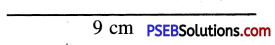# PSEB 4th Class Maths Solutions Chapter 5 Measurement Ex 5.4

Punjab State Board PSEB 4th Class Maths Book Solutions Chapter 5 Measurement Ex 5.4 Textbook Exercise Questions and Answers.

## PSEB Solutions for Class 4 Maths Chapter 5 Measurement Ex 5.4

Question 1.
Draw a line segment by joining the given points and measure their lengths: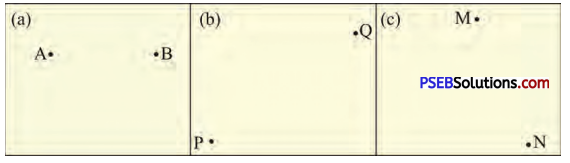Solution:
(a)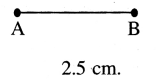(b)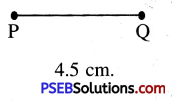(c)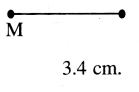Question 2.
Draw line segment of given lengths:
(a) 5 cm
Solution: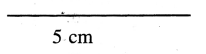(b) 8 cm
Solution: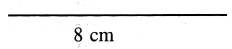(c) 6 cm
Solution: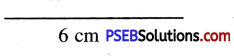(d) 2 cm
Solution: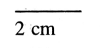(e) 7 cm
Solution: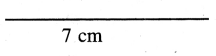(f) 9 cm
Solution: